## Saturday, November 06, 2010

### setiQuest Lagrange-4

The baudline signal analyzer looked at the setiQuest Lagrange-4 data sets from 1420, 2008, and 3991 MHz. The Lagrangian points are locations in space that are in gravitational equilibrium. The L4 and L5 points are stable orbits which would make them an ideal place to store an "object" for a very long time. Another thought is that objects may tend to naturally collect at the L4 and L5 points. The Sun-Earth L4 point was the target of this setiQuest observation.

The following command line was used to stream the Lagrange-4 data files into a prototype version of baudline:

cat 2010-10-08-Lagrange-4_3991_3-8bit-* | ./baudline -session proto -stdin -format s8 -channels 2 -quadrature -samplerate 8738133 -pause -utc 0

1420 MHz
The frequency of interstellar Hydrogen. Here is the Average spectral display: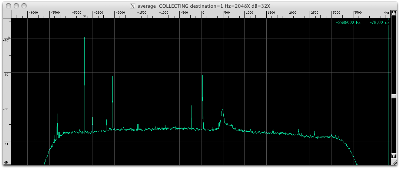Here is the signal at 1420 - 2.682582 = 1417.317418 MHz decimated by 4096. Note Hz=2X.This unusual signal jumps around making a drift measurement difficult. Four noise blobs of 33 Hz width that have some horizontal spectrum structure are present. The two middle blobs are about 100 seconds in duration and they appear to have different center frequencies.

Listen to this modulated signal in the following video. Select 720p HD and fullscreen for the best resolution.

Here is the signal at 1420 - 2.045546 = 1417.954454 MHz decimated by 4096. Note Hz=2X.This unusual wandering signal looks a lot like a 4X zoomed out version of the signal seen above at -2682582 Hz. The signal has a width of 8 Hz and drifts +19 Hz from the start to the end but it's motion looks more oscillatory.

Listen to this modulated signal in the following video. Select 720p HD and fullscreen for the best resolution.

Here is the chunk of bandwidth from 1420.029867 to 1420.032000 MHz.Three drifting signals are visible and each will be investigated below. Note that these three signals are about -460 kHz left of Hydrogen which has been a popular location for previous setiQuest signals of interest.

Here is the first signal at 1420 + 0.030525 = 1420.030525 MHz decimated by 4096. Note Hz=4X.A drifting random walk with a +107 Hz / 603 seconds = +0.177 Hz/sec drift rate. The lower half has an oscillatory drift shape with an 87 second period.

Here is the second signal at 1420 + 0.031453 = 1420.031453 MHz decimated by 4096. Note Hz=8X.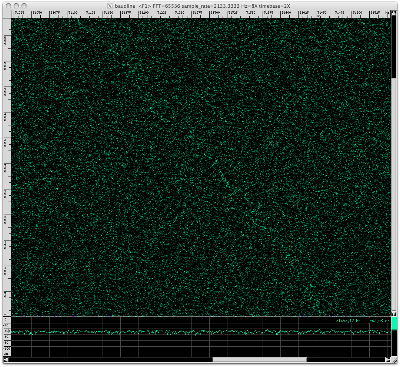This faint drifting random walk has a drift rate of +342 Hz / 603 seconds = +0.567 Hz/sec.

Here is the third signal that is at 1420 + 0.031681 = 1420.031681 MHz decimated by 4096.This wandering random walk is drifting at a net +17.1 Hz / 603 seconds = +0.0284 Hz/sec rate. Closer inspection by zooming into the time axis shows distinct frequencies that move by deltas of ±0.8 Hz. Using baudline's periodicity bar tool an extremely repetitive 3.753 symbol/second rate was measured. This looks very similar to the FSK-like zigzag modulation that was seen in the Kepler-4b redux analysis. That modulated signal was -483 kHz to the left of Hydrogen's corrected center of mass. This signal is about -460 kHz to the left of Hydrogen.

Listen to the 1420.031681 MHz modulated signal in the following video. Select 720p HD and fullscreen for the best resolution.

Here is the signal at 1420 + 0.036009 = 1420.036009 MHz decimated by 512.This fairly constant non-stationary signal is drifting at +169 Hz / 603 seconds = +0.280 Hz/sec. This interesting thing about this about this signal is its slight wiggles and small discontinuous jumps in frequency. Since the decimation rate was only 512 these fluctuations are actually much greater when compared to the other spectrograms in this blog post.

Here is the chunk of bandwidth from 1420.029867 to 1420.032000 MHz.Again, three drifting signals are visible. We will zoom in to each signal to investigate further and measure its unique characteristics.

Here is the first signal at 1420 + 0.039448 = 1420.039448 MHz decimated by 4096. Note Hz=2X.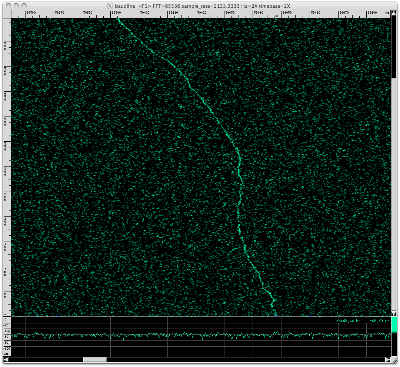Strange oscillating drift shape with a +56.0 Hz / 603 seconds = +0.0929 Hz/sec drift rate.

Here is the second signal at 1420 + 0.040146= 1420.040146 MHz decimated by 4096. Note Hz=4X.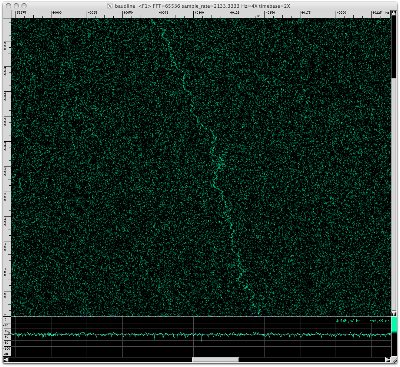A drifting random walk whose drift has oscillatory as well as random elements. The net drift rate is +75.5 Hz / 603 seconds = +0.125 Hz/sec.

Here is the third signal at 1420 + 0.040632 = 1420.040632 MHz decimated by 4096. Note Hz=2X.An exact measurement is difficult but this weaker drifting random walk is moving at roughly -20.3 Hz / 462 seconds = -0.0439 Hz/sec.

Here is a signal at 1420 + 0.045422 = 1420.045422 MHz decimated by 4096.This narrow band noise signal is almost stationary with a -2 Hz / 603 seconds = -0.003 Hz/sec drift rate. Closer inspection of the lower third reveals alternating FSK-like blips with a 1.2 Hz delta and a repetitive periodicity of 7.1 seconds. The consistent spacing and periodicity suggest that this is not statistical noise.

Here is a signal which is -75 kHz left of Hydrogen peak at 1420 + 0.415107 = 1420.415107 MHz decimated by 4096. Note Hz=2X.Looks like scatter noise. Could be caused by multipath. Measuring drift rate is not possible.

Here is the very strong signal on the far right of the spectrum edge at 1420 + 4.315733 = 1424.315733 MHz decimated by 4096. Note Hz=4X.This is a stationary pulsing tone. Several faint harmonic lines are visible on both sides of the main tone. Here is the Average display with the frequency axis zoomed out one notch.The harmonics are at ±50, ±73, ±120, and ±200 Hz which are all very suspicious values. Here is a spectrogram with the I&Q channels using the Histogram transform.The strong pulsing tone, ±50 and ±73 Hz harmonics, and wandering I&Q Histogram spectrogram were all seen in the Kepler-4b redux analysis with the -2422400 Hz signal. I suspect both were caused by the same distortion phenomena that is internal to the ATA. The Kepler-4b redux dataset was recorded almost 5 months prior.

2008 MHz
sqrt(2) * 1420 = 2008.This faint noise signal shifts -12 Hz in a fairly fast transition that last 101 seconds. At first this signal appears to be stationary but each section has a slight -0.0087 Hz/sec drift rate. A higher resolution signal is required to be certain but this has a classic Doppler flyby shape I often see with acoustic recordings of planes and helicopters.

Here is the signal at 2008 - 3.160508 = 2004.839492 MHz.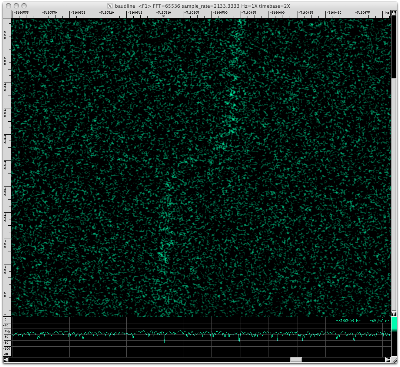This signal looks like the two-tone Doppler flyby above but it is +6400 Hz to the right in frequency and it has more well defined pulsing. Using baudline's periodicity bars a 26.3 second pulse rate was measured. The pulses before the flyby are also time aligned to those after.

Here is the signal at 2008 - 2.251751 = 2005.748249 MHz decimated by 4096. Note Hz=2X.This strange looking signal is about 2 Hz wide and it switches between two different linear drift rates. The net drift rate of -53 Hz / 579 seconds = -0.092 Hz/sec is composed of a slower -0.026 Hz/sec rate and a faster -0.21 Hz/sec rate. Baudline's periodicity bars show that the pulsing globs line up nicely with a 51 second periodicity spacing.

Here is the signal at 2008 - 2.184533 = 2005.815467 MHz.Stationary signal with zero drift rate. Repeated pulsing groups suggest signal could contain modulated content.

Here is the signal at 2008 - 2.177574 = 2005.822426 MHz decimated by 4096. Note Hz=2X.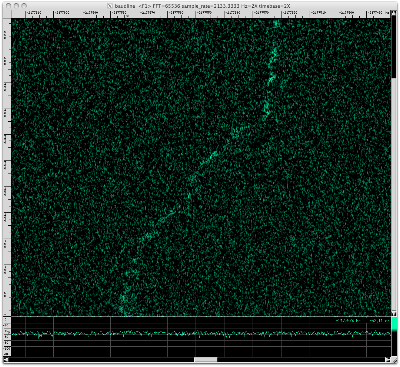This is a weaker version of the -2251751 Hz signal we saw above.

Here is the signal at 2008 - 2.103398 = 2005.896602 MHz decimated by 4096. Note Hz=2X.Another weaker version of the signal we saw above.

Here is the signal at 2008 - 1.948203 = 2006.051797 MHz decimated by 4096. Note Hz=8X.Narrow band noise-like pulses. Drift rate of -110 Hz / 271 seconds = -0.406 Hz/sec. The pulse bursts line up with a 87 second periodicity.

Here is the signal at 2008 + 1.094480 = 2009.094480 MHz decimated by 4096. Note Hz=8X.Drift rate of 240 Hz / 579 seconds = 0.415 Hz/sec. The previous signal above had a similar drift rate but looked completely different. Measured a periodicity of 52 seconds which matches that seen in the -2251751 Hz signal. That signal had the same periodicity but a quarter of the drift rate. It is interesting that this signal shares characteristics with two different signals, they are clearly related but not exactly.

3991 MHz
This frequency is expected to be a "bad band" filled with lots of C-band satellite signals.

This signal's bandwidth is 2.5 MHz wide. The spectrogram and Average spectrum look to be filled with an incredible number of signals. Let's zoom into the frequency axis of the Average spectrum:

There are thousands of narrow well defined signals everywhere you look in the spectrum Let's zoom in some more:

Using baudline's fundamental Hz measurement window the delta between peaks was accurately measured to be 1267.226 Hz. That is an interesting value because ...

Next let us decimate by 4096 and zoom into the strongest signal peak at 1502 kHz.

Slowly drifting to the left with a general drift rate of -0.91 Hz / 295 seconds = -0.0033 Hz/second. The signal erratically jumps between several discrete frequencies with deltas of {∆ 0.5, 1.0, 1.4, 3.2 Hz}. This signal is clearly modulated. Using the periodicity bars a symbol rate of 1 / 2.783 seconds = 0.359 symbols/second is measured.

Randomly spot checking 20 of the tone peaks reveals the same shape with varying amplitudes. So I believe that there are thousands of copies of this same signal. This could be caused by AM modulation, distortion in the ATA signal chain, or it could be a unique characteristic of this modulation scheme.

Listen to this modulated signal in the following video. Select 720p HD and fullscreen for the best resolution.

Something else extremely interesting is going on with this signal. Here is the autocorrelation spectrogram of the same signal seen above decimated by another factor of 64 for a total decimation ratio of 262144.The two patterns of interest are the strange shapes in the middle the 6+ holes near the bottom. They signify a more complex structure than you would expect from a drifting random walk. Similar shapes and holes were also seen in several other baudline-setiQuest analyses such as the two Kepler-4 blog posts.

Here is the spectrogram of the blip Fourier transform in phase space.Look along the vertical center-line (arrow) and notice the evenly spaced black holes near the bottom. Their periodic spacing measurement is 5.81 seconds for 7 consecutive major feature changes. This distribution is too uniform to be a statistic fluke. They signify 180º phase shifts which suggest a BPSK like modulation.

Here is a plot of the Autocorrelation transform using the Average window of the full bandwidth signal.The evenly spaced spikes represent that a repetitive pattern is present. This shape suggests the signal is direct-sequence spread spectrum (DSSS) and possibly CDMA. The spacing between autocorrelation peaks is 1 / 789.07 us = 1267.3 Hz which interestingly is within 0.1 Hz of the spectral measurement above.
The vertical dash-dot patterns represent changing bits (groups actually). Any common patterns you see are likely repeating header or idle sequences.

Conclusion
The Lagrange-4 datasets contained an incredible number of unique signals. Drifting random walks were a common theme in the 1420 MHz band while 2008 MHz was mostly populated with variations of a wider band Doppler flyby signal. Many of these signals had modulated features. Determining the source of these unknown signals is not really possible with the available information. This fairly sums up the challenge of SETI; detecting weak signals is easy, determining extraterrestrial origin is difficult.

The 3991 MHz band contained one 2.5 MHz wide signal that I suspect is CDMA. In the comments below a reader named Martin posted some extremely interesting information about the STEREO (Solar TErrestrial RElations Observatory) NASA satellites at the L4 and L5 positions (see plot below).

Martin mentions STEREO having a 633.245 bps data rate at 8.4 GHz. Twice this data rate is 1266.490 bps which is very close to the 1267.226 Hz spectral value and the 1 / 789.07 us = 1267.3 Hz autocorrelation rate I measured. The average error is 0.75 Hz which seems slightly greater than the accuracy level I felt baudline measured but since this signal is wiggling around by almost ±2 Hz it is in the realm of being a plausible match. Explaining how such a low baud rate signal gets down-converted by 4.4 GHz and expanded into a CDMA-like 2.5 MHz wideband signal is more difficult. In any event, this match is potentially an amazing discovery that should help in understanding the distortion characteristics of the ATA. Thank you Martin.

There were too many signals in the Lagrange-4 datasets to be able provide the quality of coverage each signal deserved. This blog post is the last time that I will attempt an exhaustive analysis of all the signals in a data set. It is a quantity vs. quality trade-off. Future baudline-setiQuest blog posts will focus on a single feature of interest. I also plan on incorporating more video clips so let me know what you think of them in the comments and how they might be more useful.

Unknown said...

Baudline -

After some googling I can only find one spacecraft currently at Earth-Sun L4. That is the STEREO observatory (its twin at L5). Some downlink characteristics at:

http://www.amsat.org/amsat/articles/g3ruh/127.html

Based on the quote below it sounds like the main accessible signal is in the GHz neighborhood. So I read that as nothing to do with this?

--------------------------------
spacecraft transmit 63 watts to a 1.1m dish at 8.4 GHz RHCP, an e.i.r.p. of approximately 85 dBm. The range at present (2010 Oct) is about 195M km, increasing very slowly.

Signal format

The data signal is square-wave Manchester-encoded binary data of 633 bps, <15,6> convolutionally encoded to 3.8 ksym/s and phase-modulated onto the spacecraft carrier. The modulation index is +/-0.6 radian, so 68% of the signal power is in the carrier, 32% given to the data.

* Data Rate: 633.245 bps
* Symbol rate: 3799.472 sym/s
* Bytes per block: 1119 bytes incl. crc and sync
* Bits/block: 8952 bits
* Symbols/block: 53712 sym
* Block period: 14.137 s

baudline said...

Martin, thank you for the amazing sleuthing work. Stereo's 633 bps data rate looks to be a good match for the 1267 Hz spectral and autocorrelation values that were measured at 3991 MHz. Why this modulated signal has been down-converted by 4.4 GHz and spread out to a 2.5 MHz bandwidth is a good question. See the recently updated videos and conclusion section for more info.

baudline said...

I added a plot in the Conclusion section that shows the positions of STEREO A & B for the date of the signal collection. They are clearly near the L4 & L5 positions.

It is still a mystery how STEREO's 8.4 GHz signal could get down-converted to 3991 MHz by the ATA. Maybe it is some sort of sideband / distortion / artifact? It would be interesting to hear the opinion from someone at SETI Institute about this.